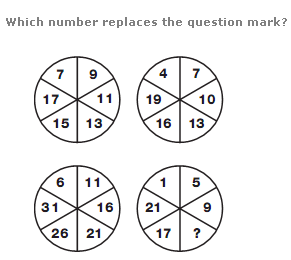# Missing Number puzzles: Interviews, Placements and Competitive Exams#### MISSING NUMBER PUZZLESAnswer : 6 Explanation : Looking at the diagram in rows, the central circle equals half the sum of the numbers in the other circles to the left and right of the centre.Answer : 19 Explanation : As you move diagonally down, numbers follow the sequence of Prime Numbers.

Attempt the below quiz to know your thinking skills(POST ANSWERS IN THE COMMENTS WITH QUESTION NUMBER):

Question 1:Question 2:Question 3:Question 4:Question 5:Answers with Explanation:

1. 6; Looking at the diagram in rows, the central circle equals half the sum of the numbers in the other circles to the left and right of the centre.
2. 19; As you move diagonally down, numbers follow the sequence of Prime Numbers.
3. 39; Working from top to bottom, double each number and subtract 1, then 2, then 3 etc.
4. 40,  Moving from left to right, numbers increase by 2,3,4 and 5.
5. 13, In each circle, starting at the top left segment, numbers increase, as you move clockwise, by 2 for the upper left circle, 3 for the upper right, 4 for the lower right and 5 for the lower left.
Don't miss out!
Subscribe To Our Newsletter

Learn new things. Get an article everyday.

Invalid email address
Give it a try. You can unsubscribe at any time.